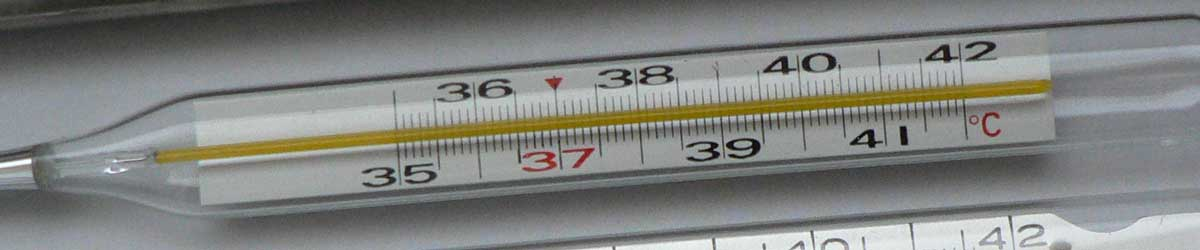# Newton to Fahrenheit Conversion

Enter the temperature in Newton below to get the value converted to Fahrenheit.

Results in Degrees Fahrenheit:1 °N = 37.454545 °F

## How to Convert Newton to FahrenheitConvert degrees Newton to degrees Fahrenheit with this simple formula:

degrees Fahrenheit = [°N] × 60 / 11 + 32

Insert the [°N] temperature measurement in the formula and then solve to find the result.

For example, let's convert 50 °N to degrees Fahrenheit:

50 °N = ( 50 × 60 / 11 + 32 ) = 304.727273 °F

## Newton

The Newton scale is a temperature scale that sets a fixed zero point equal to the freezing point water. The scale uses both arithmetic progression and geometric progression. The scale defines 18 reference points and the corresponding "degrees of heat" and geometric progressions.

Newton can be abbreviated as N; for example, 1 degree Newton can be written as 1 °N.

## Fahrenheit

The Fahrenheit scale is a temperature scale that defines the melting point of water as 32 degrees and the boiling point of water at 212 degrees. There are 180 intervals between 32 °F and 212 °F, each corresponding to one degree.

The degree Fahrenheit is a US customary and imperial unit of temperature. Fahrenheit can be abbreviated as F; for example, 1 degree Fahrenheit can be written as 1 °F.

### Important Temperatures In Degrees Fahrenheit

TemperatureDegrees Fahrenheit
Absolute Zero-459.67 °F
Freezing Point of Water32 °F
Triple Point of Water32.018 °F
Boiling Point of Water212 °F

## Newton to Fahrenheit Conversion Table

Newton measurements converted to Fahrenheit
Newton Fahrenheit
-50 °N -240.73 °F
-40 °N -186.18 °F
-30 °N -131.64 °F
-20 °N -77.09 °F
-10 °N -22.55 °F
-5.8667 °N -0.000002 °F
0 °N 32 °F
10 °N 86.55 °F
20 °N 141.09 °F
30 °N 195.64 °F
40 °N 250.18 °F
50 °N 304.73 °F
60 °N 359.27 °F
70 °N 413.82 °F
80 °N 468.36 °F
90 °N 522.91 °F
100 °N 577.45 °F
110 °N 632 °F
120 °N 686.55 °F
130 °N 741.09 °F
140 °N 795.64 °F
150 °N 850.18 °F
160 °N 904.73 °F
170 °N 959.27 °F
180 °N 1,014 °F
190 °N 1,068 °F
200 °N 1,123 °F
300 °N 1,668 °F
400 °N 2,214 °F
500 °N 2,759 °F
600 °N 3,305 °F
700 °N 3,850 °F
800 °N 4,396 °F
900 °N 4,941 °F
1,000 °N 5,487 °F

## References

1. National Oceanic and Atmospheric Administration National Weather Service , Fahrenheit, https://w1.weather.gov/glossary/index.php?word=fahrenheit